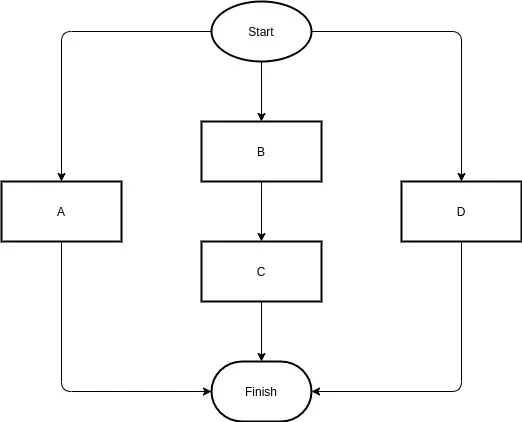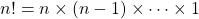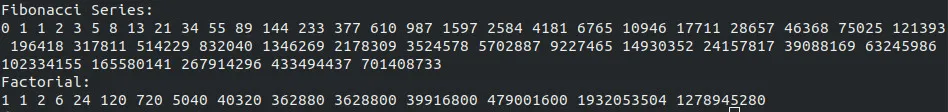# Programming - Parallel Sections in OpenMP[Image 1]

## Introduction

Hey it's a me again @drifter1!

Today we continue with the Parallel Programming series about the OpenMP API. I highly suggest you to go read the previous articles of the series, that you can find by the end of this one. Today we will get into how we define Parallel Sections.

So, without further ado, let's get straight into it!

## Quick Recap

A parallel region is defined using a parallel region construct:

``````#pragma omp parallel
{
/* This code runs in parallel */
}``````
and can be configure using the following clauses:
• Conditional parallelism - if(condition) clause
• Default data sharing - default(...) clause
• List of private variables - private(...) clause
• List of shared variables - shared(...) clause
Parallel For Loops are defined by using the following syntax:
``````int i;
#pragma omp parallel for private(i)
for(i = 0; i < N; i++){
...
}``````
and can be configured - in addition to the previously mentioned clauses - by using the following clauses:
• List of private variables with initialization to shared variable - firstprivate(...) clause
• List of private variables with assignment towards shared variable in last iteration - lastprivate(...) clause
• Define how iterations are divided amongst threads - schedule(...) clause
• Specify if threads should be synchronized by a barrier or not - nowait(...) clause
• Specify if iterations should be executed as in a serial programm - ordered(...) clause
• Specify how many nested loops should be collaped in to one large iteration space - collapse(...) clause
• Specify if the compile should try to reduce the number of iterations - reduction(...) clause

## Parallel Section(s) Construct

### Why Sections?

With for loops we saw how we can divide the various iterations among threads to execute them faster when parallelization is possible. What if there are specific sections of code that can be run in parallel whilst other can't?

Let's take the following flowchart for example:[Custom Figure using draw.io]

From the chart we understand that B and C have to be executed in sequential order (B → C).

If we somehow could indicate that B and C should be executed sequentially, then A, B → C and D could be executed in parallel!

That's were sections come into play...

### Section(s) Construct

A sections construct (with s!) is a directive that is used to define non-iterative work-sharing among threads in a team (that is already defined using a parallel section).

Independent section constructs (without s!) are nested within the sections construct. Each of these sections is executed once by a thread in the team and different sections might be executed by different threads. Its a matter of how quickly a thread manages to execute a section and how the implementation defines such behavior.

A sections construct with nested section directives is defined as:

``````#pragma omp sections [clause ...]
{
/* run in parallel by all threads of the team */
#pragma omp section
{
/* run once by one thread */
}
#pragma omp section
{
/* run once by one thread */
}
...
}``````
To define sections and create a team of threads, at the same time, we can use the following shortcut:
``#pragma omp parallel sections``
For example, for the flow-chart example we would write:
``````#pragma omp parallel sections num_threads(3)
{
#pragma omp section
{
/* Code for Work A */
}
#pragma omp section
{
/* Code for Work B */
/* Code for Work C */
}
#pragma omp section
{
/* Code for Work D */
}
}``````
That way A, B → C and D will be executed in parallel by 3 threads, with each one taking one section (possibly).

It's worth noting that a sections construct cannot be used inside of another work-sharing construct (like the parallel for that we saw). To implement more advanced work-sharing we have to use tasks, that we will cover later on in this series.

### Single (Serial Section) Construct

A similar construct that is quite useful when only one section inside a parallel region has to be executed by one thread.

Instead of creating a nested section inside of sections to write that code, we can just use a single construct.

The syntax of such a construct is simply:

``#pragma omp single``
If only thread 0 (master thread) should run this section then we can also use:
``#pragma omp master``
Of course both of them make sense only when used inside of a parallel region.

### Section Clauses

The following clauses can be used to configure sections:

• list of private variables - private(...), firstprivate(...), lastprivate(...)
• if sections should be reduced by the compiler (when possible) - reduction(...)
• if threads should be synchronized by a barrier or not - nowait(...) clause

## Example Program

Let's execute a calculation of the Fibonacci series and the Factorial (!) in parallel by using sections.

The Fibonacci series is defined as:The Factorial is calculated as:It's worth noting that 0! = 1.

### Fibonacci

To calculate the Fibonacci series in C we write the following function:

``````void fibonacci(int A[]){
int i;
A = 0;
A = 1;
for(i = 2; i < N; i++){
A[i] = A[i - 1] + A[i - 2];
}
}``````

### Factorial

To calculate the Factorial in C we write the following function:

``````void factorial(int A[]){
int i;
A = A = 1;
for(i = 2; i < M; i++){
A[i] = i * A[i - 1];
}
}``````

### Main

In the main function we simply define two arrays with size N and M (global `#define`) and create a parallel section to execute the two functions in.

``````#include <omp.h>
#include <stdio.h>
#include <stdlib.h>
#define N 45 /* Fibonacci limit */
#define M 15 /* Factorial limit */
... functions ...
int main(){
unsigned int fib[N];
unsigned int fac[M];
int i;
/* Parallel Sections */
#pragma omp parallel sections
{
/* Calculate Fibonacci Series */
#pragma omp section
{
fibonacci(fib);
}
/* Calculate Factorial */
#pragma omp section
{
factorial(fac);
}
}
/* print arrays */
printf("Fibonacci Series:\n");
for(i = 0; i < N; i++){
printf("%u ", fib[i]);
}
printf("\n");
printf("Factorial:\n");
for(i = 0; i < M; i++){
printf("%u ", fac[i]);
}
printf("\n");
return 0;
}``````

### Output

Running the program for N = 45 and M = 15 we get:which are the values that we expected...

There are of course better ways to use sections, but running two different operations in parallel is also not that bad(!)

## RESOURCES:

### Images

1.## Final words | Next up

And this is actually it for today's post!

Next time we will get into Atomic Operations and Critical Sections...

See ya!Keep on drifting!

H2
H3
H4
3 columns
2 columns
1 column
1 Comment